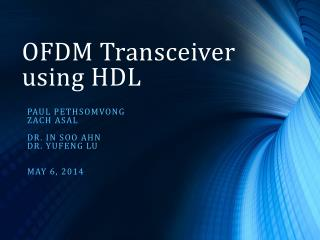DownloadDownload PresentationOFDM Transceiver using HDL

# OFDM Transceiver using HDL

Download Presentation## OFDM Transceiver using HDL

- - - - - - - - - - - - - - - - - - - - - - - - - - - E N D - - - - - - - - - - - - - - - - - - - - - - - - - - -
##### Presentation Transcript

1. OFDM Transceiver using HDL Paul pethsomvong Zach asal Dr. in sooahn Dr. yufenglu May 6, 2014

2. Outline • Project Overview • Project Goal • Project Description • Orthogonal Frequency Division Multiplexing (OFDM) • Communication Channel Effect • Specifications • Results • Conclusion • References

3. Project Overview • Implements an Orthogonal Division Multiplexing (OFDM) communication system. Figure 1: Block Diagram

4. Project Goal • To implement a complete OFDM system using field programmable gate arrays (FPGA) and VHDL hardware description language. The OFDM system can be reconfigurable.

5. Project Description • The project is divided into three stages. • Stage 1: MATLAB/Simulink • Stage 2: FPGA board implementation with channel effects

6. What is OFDM • Orthogonal frequency division multiplexing (OFDM) is a technique for transmitting digital data by using a large number of modulated carriers with sufficient frequency spacing so that the carriers are to be orthogonal.  • OFDM involves encoding data on multiple carrier frequencies, all of which are orthogonalto each other

7. OFDM signal • g(t) is the complex envelope for OFDM signal. • fn(t) are orthogonal carriers.

8. Orthogonal

9. Complex Envelope Representation Figure 2: Complex Envelope Representaion

10. Communication Channel Effects • Attenuation • Multipath • Delay spread • Doppler spread • Multipath fading • Inter-symbol interference

11. Attenuation • Reduction in power density • Occurs as the distance from the source increases • absorption, free-space loss, refraction , etc.

12. Multipath • Delay Spread • delay spread is caused by multiple paths with varying lengths. • Doppler Spread • Caused by different movements of various multipath reflectors

13. Multipath Fading • Fading caused when different multipath reflectors move around slowly and appear and disappear. • Fading is due to the received signals cancelling each other out.

14. Inter-symbol Interference (ISI) • One symbol interferes with subsequent symbols • Each symbol spreads in time, the pulse for each symbol may be smeared into adjacent symbol slots • Causes communication to be less reliable

15. Applications & Advantages • Resistant to multipath fading • Low inter-symbol interference • Widely used in digital communication systems • Used in 802.11(a, g, n, ac), 4G, and LTE • 4G - 4th generation wireless communication • LTE- Long Term Evolution

16. FFT/IFFT • This is the most important block in the OFDM communication system. • The FFT and IFFT are implemented using the Xilinx core generator.

17. Cyclic Prefix • Trim and align data Figure 3: Cyclic Prefix

18. Equipment • Genesys FPGA board • Xilinx • Diligent PmodDA2 • Diligent PmodAD1 Figure 4: Genesys FPGA board

19. Specifications Figure 5: Specifications

20. FPGA System Clock = 100 MHz • Symbol Rate Rs = 12.5 Msps • Data rate Rb= Rs · N = 50 Mbps • Bits per symbol N = 4 • Symbol time = 1/ Rs

21. Stage 1 Simulink simulation • A single subcarrier carries data. Figure 6: 1 Frequency

22. Stage 1 Simulink simulation • Two subcarriers carry data. Figure 7: 2 frequencies

23. 32 subcarriers Figure 8: 32 frequencies

32. Simulink Model • Model contains transmitter, receiver, and a Gaussian channel model. • Channel model • Y(t) = x(t)*h(t) • where x(t) is the transmitted signal, h(t) is the impulse response of the channel, and y(t) is the received signal.

33. Simulink results Figure 9: Input and Output from Transceiver

34. Stage 2: Implementation with channel effects • The transmitter and receiver are implemented on a FPGA board using VHDL. • Analog-to-digital converter (ADC) and digital-to-analog converter (DAC) modules are included. • Channel effects degrade the overall system performance.

35. Figure 10: 16-QAM Transmitted